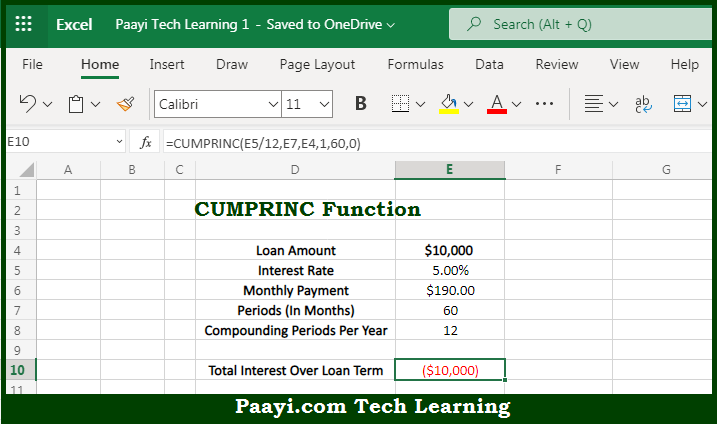# Learn How to Use Microsoft Excel CUMPRINC Function

Written by | 0 Comments | 479 Views

In this article, you will learn how to use the Microsoft Excel CUMPRINC function and its prime function in Microsoft Excel. You will also get to know the Microsoft Excel CUMPRINC function return value and syntax with the help of some examples.

Microsoft Excel CUMPRINC Function

The main purpose of the Microsoft Excel CUMPRINC function is to get the cumulative principal paid on the loan. That implies, with the help of the CUMPRINC function you can able to return the cumulative principal paid on a loan between a start period and an end period. It should be noted that you can use the CUMPRINC function to calculate and verify the total principal paid on a loan, or the principal paid between any two payment periods. So, with the help of the CUMPRINC function, you can able to get the cumulative principal paid on the loan.

Return Value of CUMPRINC Function

The return value will be the interest amount.

Syntax of CUMPRINC Function

=CUMPRINC(rate, nper, pv, start_period, end_period, type)

Where the arguments:

• rate: This is the interest rate of the period.
• nper: This is the total number of payments for the loan.
• pv: This is the present value or total values of all payments now.
• start_period: This is the first payment in the calculation.
• end_period: This is the last payment in the calculation.
• type: This can be understood as when payments are due. 0 = end of the period. 1 = beginning of the period.

How to Use Microsoft Excel CUMPRINC Function?So we know that Microsoft Excel CUMPRINC function you can able to get the cumulative principal paid on the loan. That implies, with the help of the CUMPRINC function you can able to return the cumulative principal paid on a loan between a start period and an end period. It should be noted that you can use the CUMPRINC function to calculate and verify the total principal paid on a loan, or the principal paid between any two payment periods. So, with the help of the CUMPRINC function, you can able to get the cumulative principal paid on the loan.